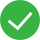# 表计算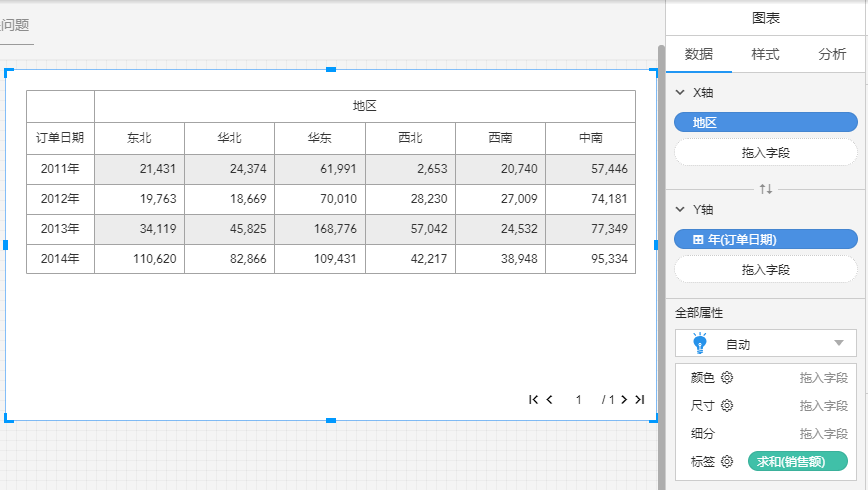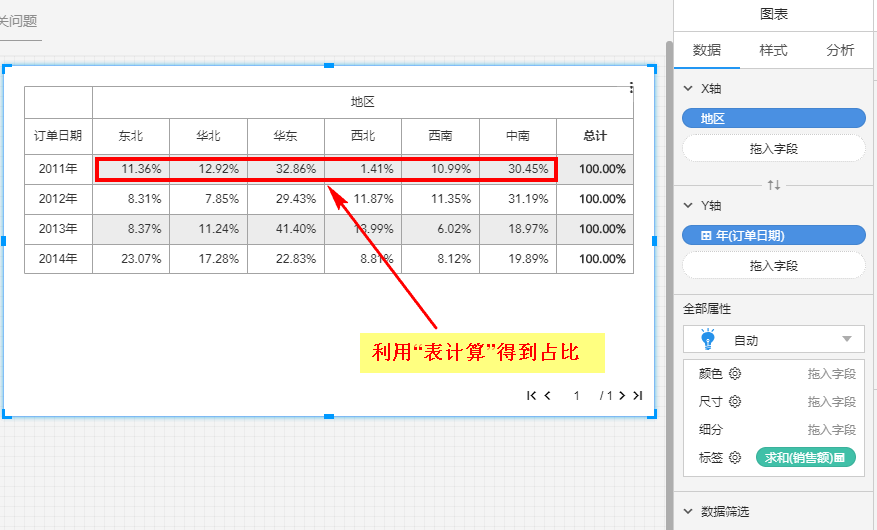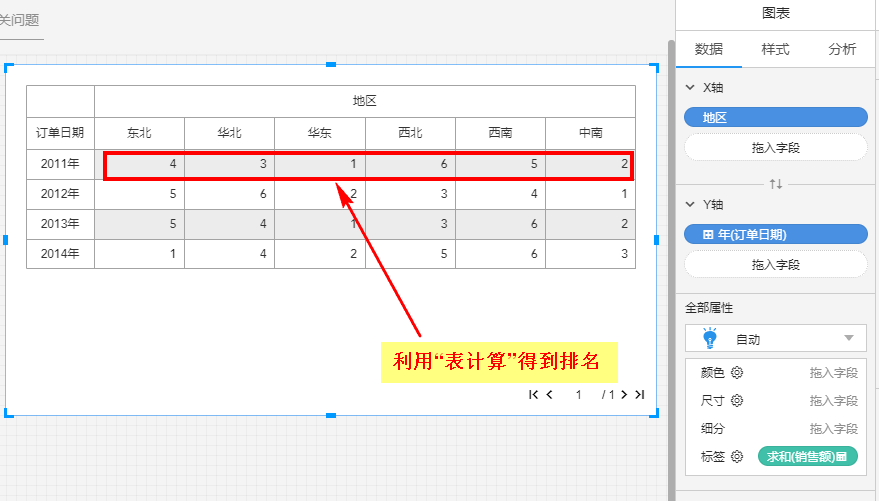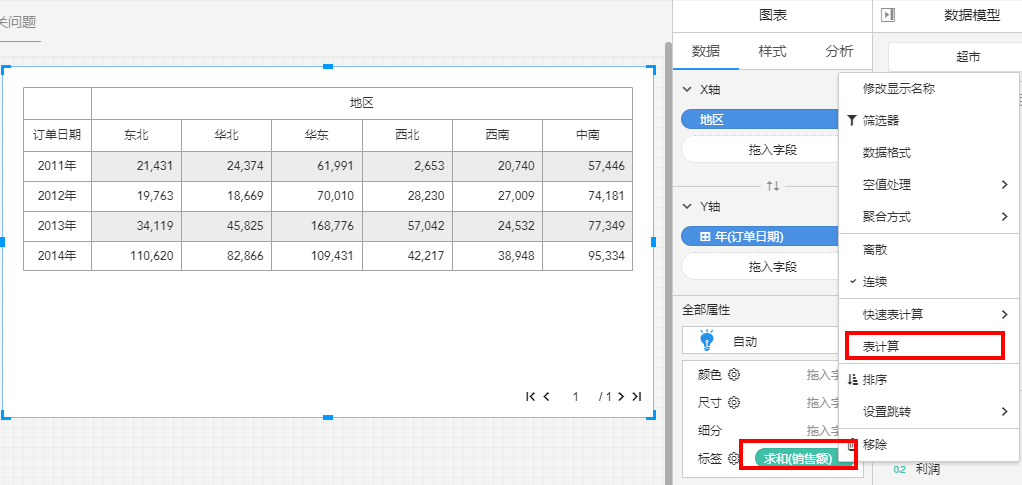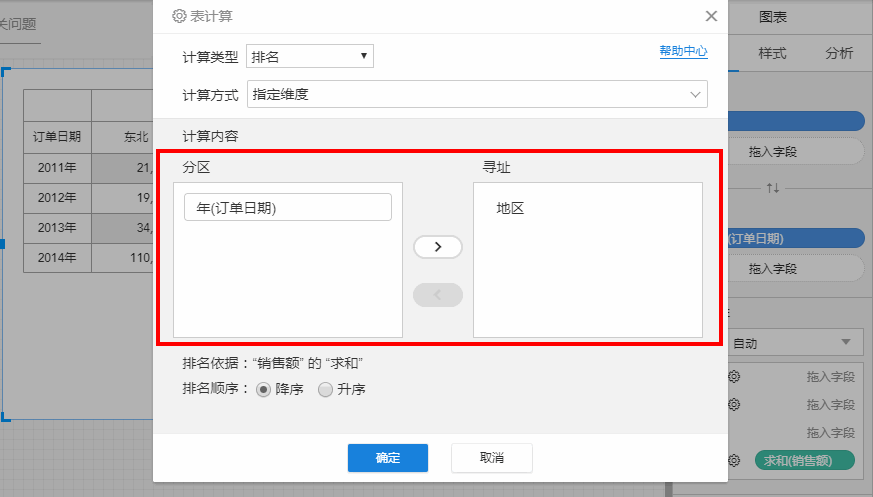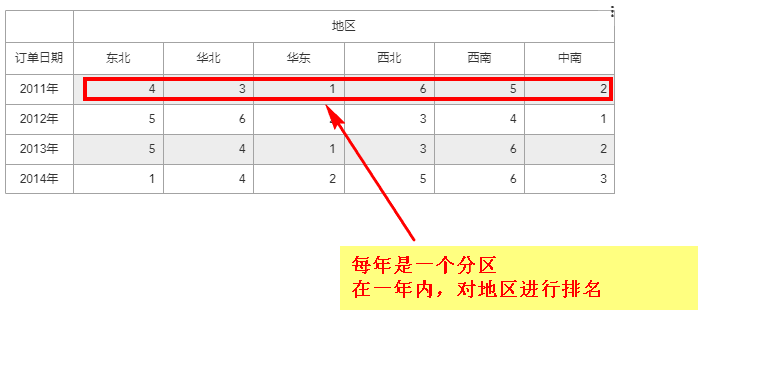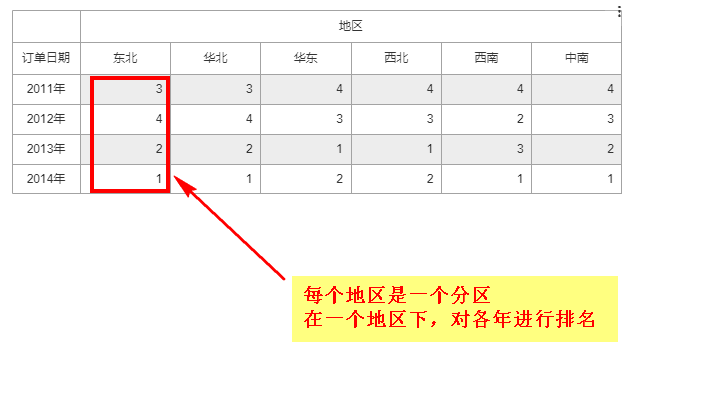## 差异

$100 = 200 - 100$


$D_x = A_x - A_{x-1}$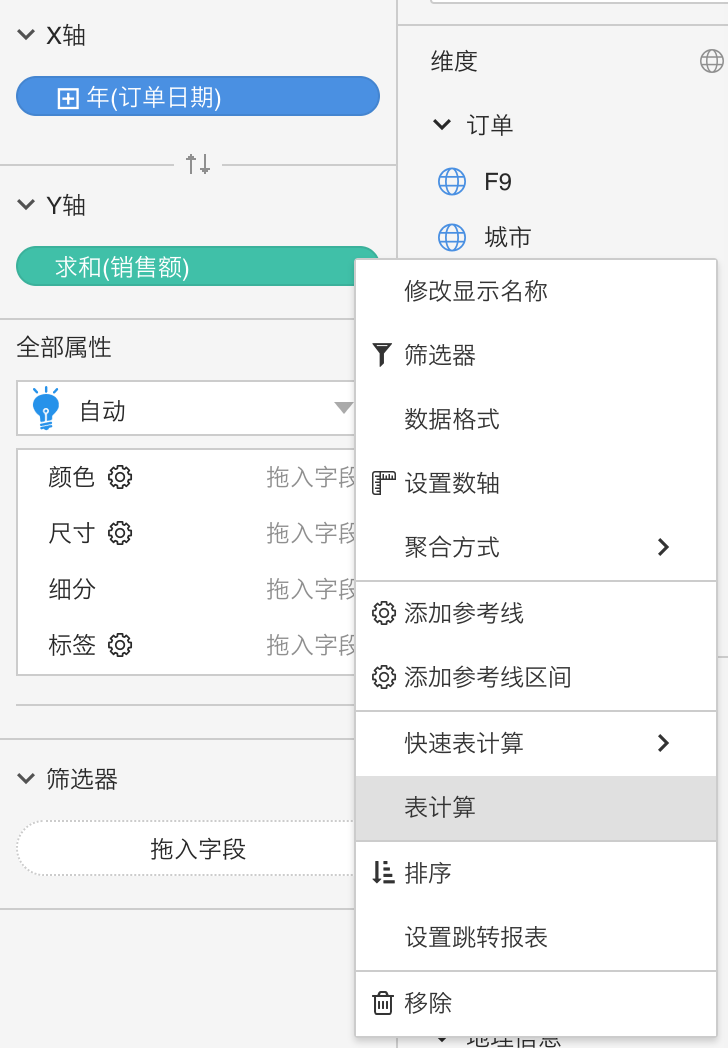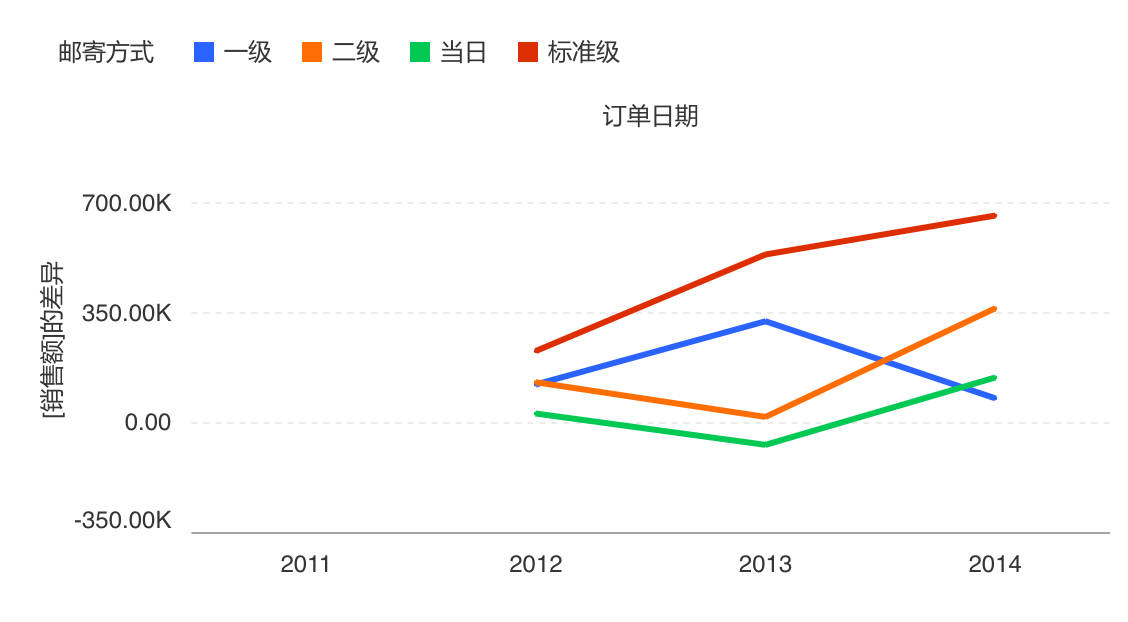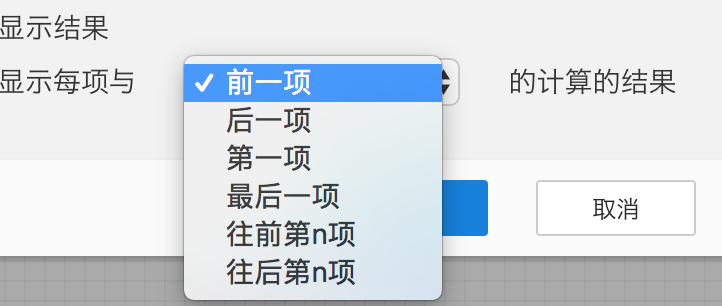## 百分比

$200\% = 200 \div100 \times 100\%$


$P_x = A_x \div A_{x-1} \times 100\%$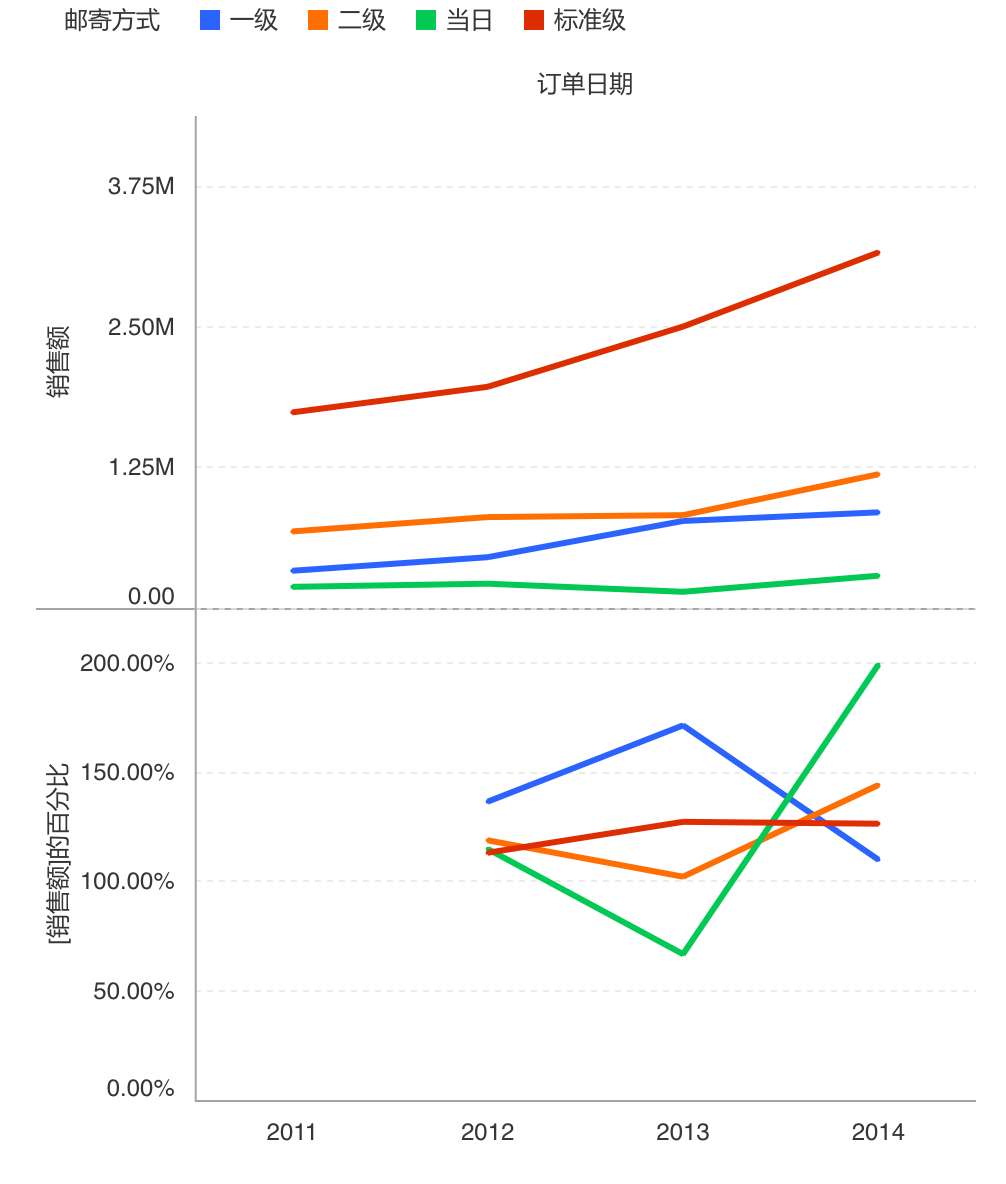## 差异百分比

$100\% = (200 - 100) \div100 \times 100\%$


$P_x = (A_x - A_{x-1}) \div A_{x-1} \times 100\%$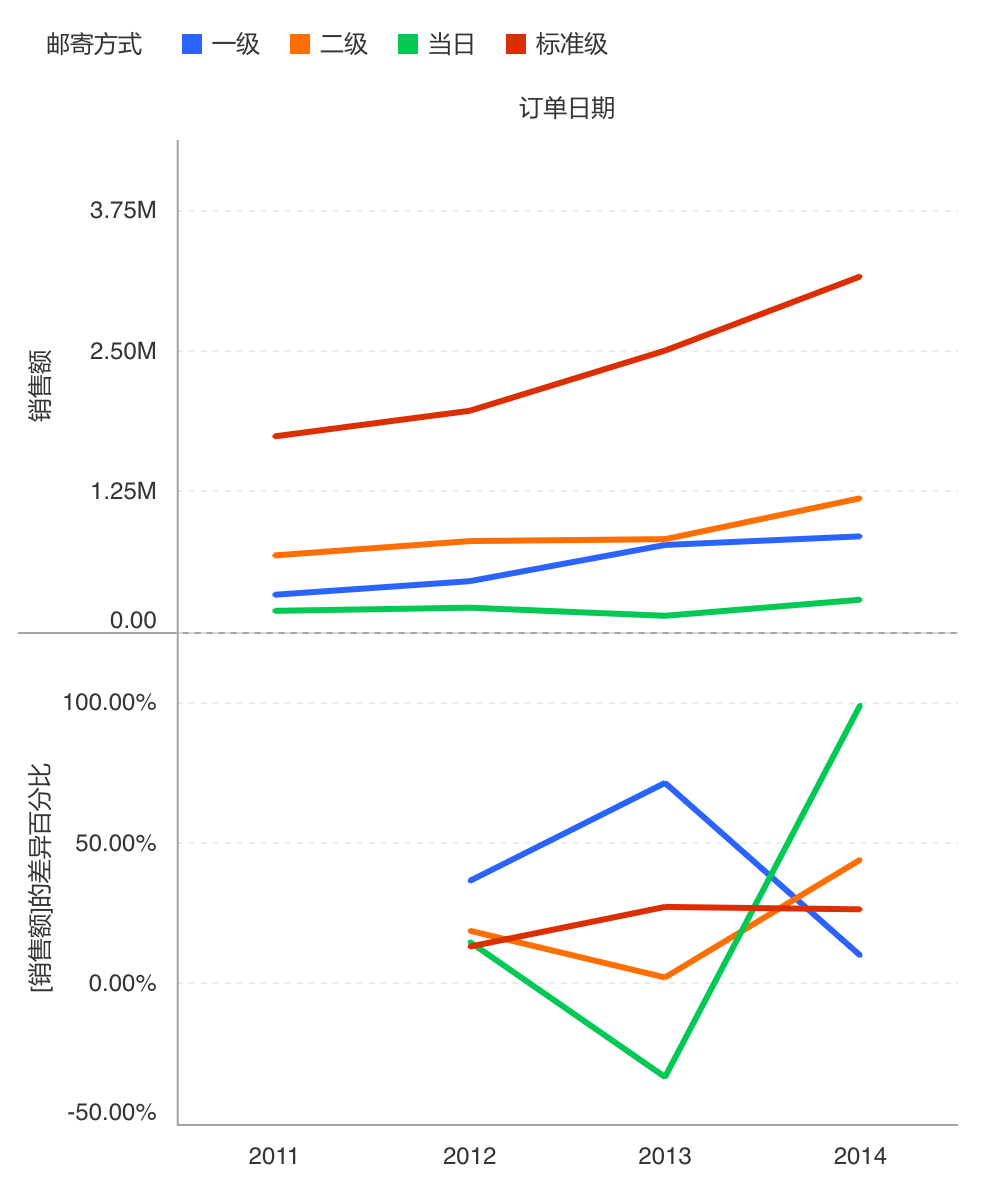## 总额百分比

$10\% = 200 \div 2000 \times 100\%$


$P_x = A_x \div \Sigma A \times 100%$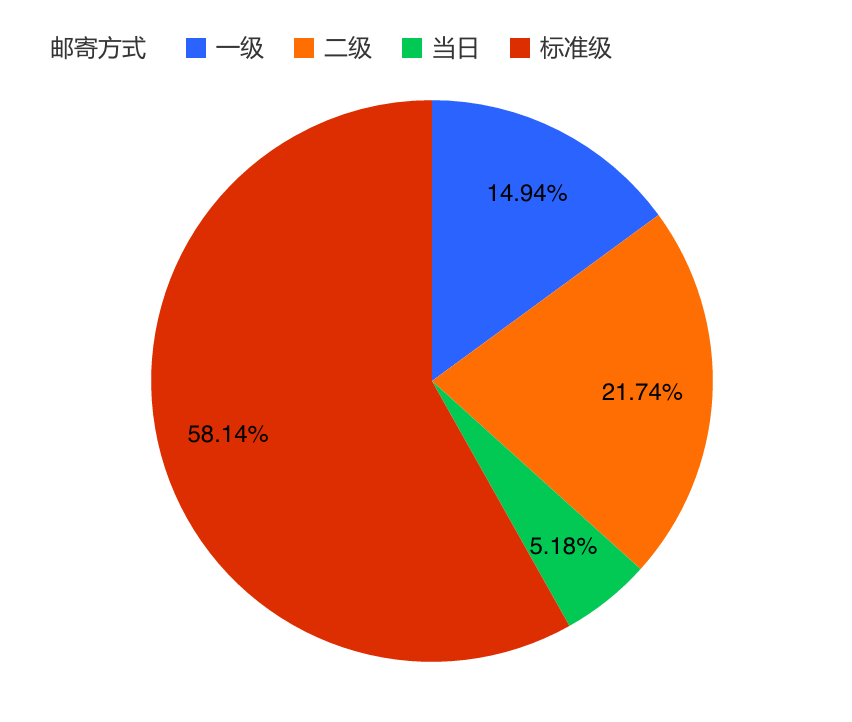## 累积和

$1000 = 100+200+300+400$


$CS_x = \Sigma_{i=0}^{x} A_{i}$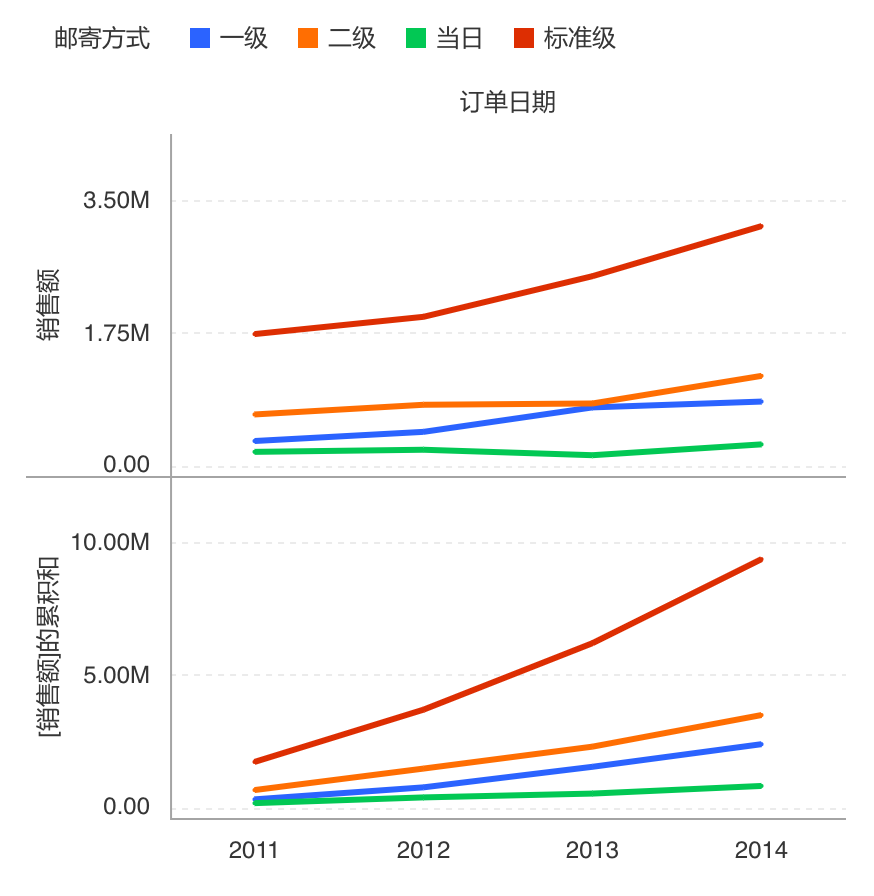## 移动计算

$400 = (200 + 400 + 600) \div 3$


$WS_x(-1,1) = AGG_{x-i}^{x+1}$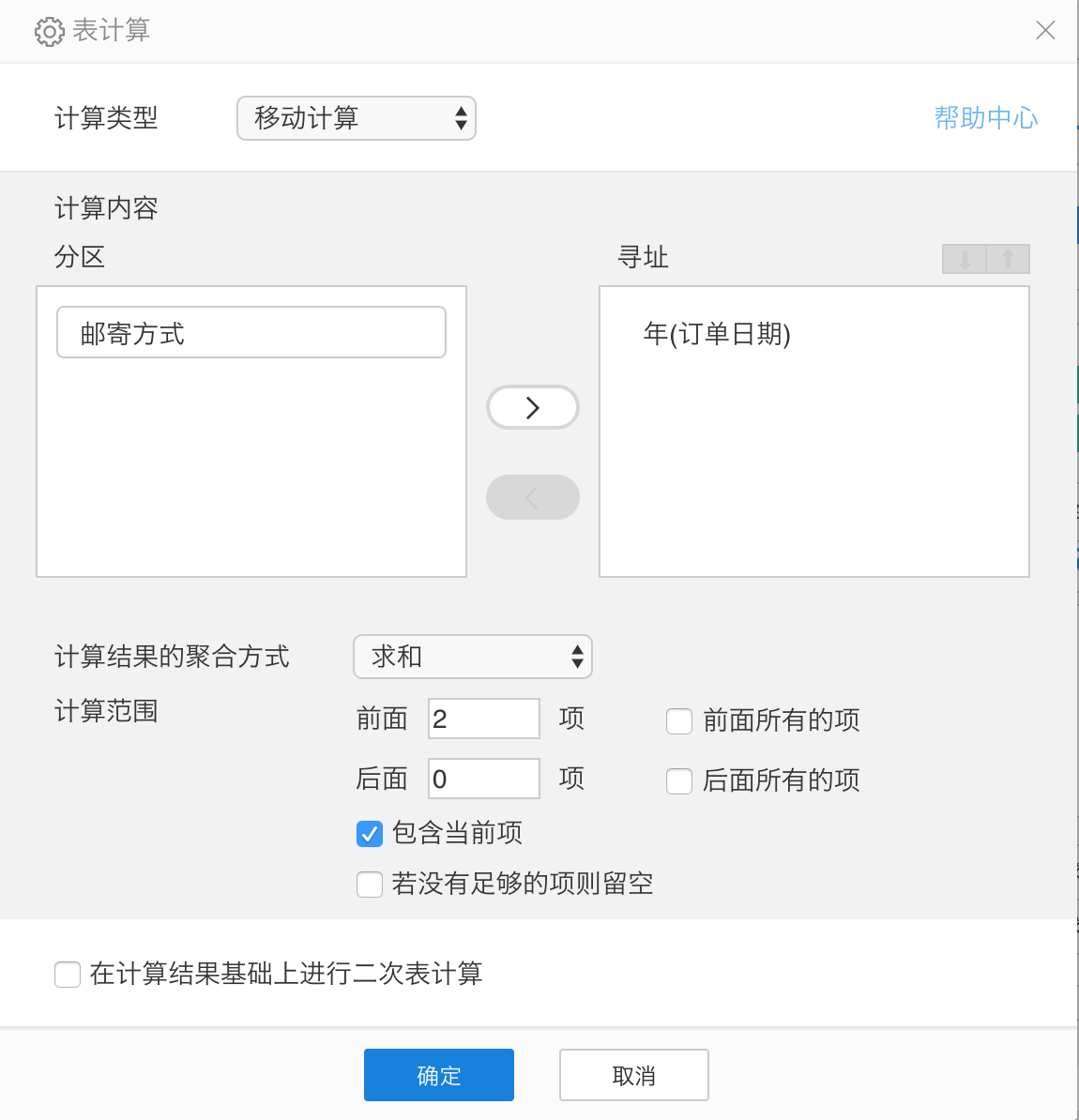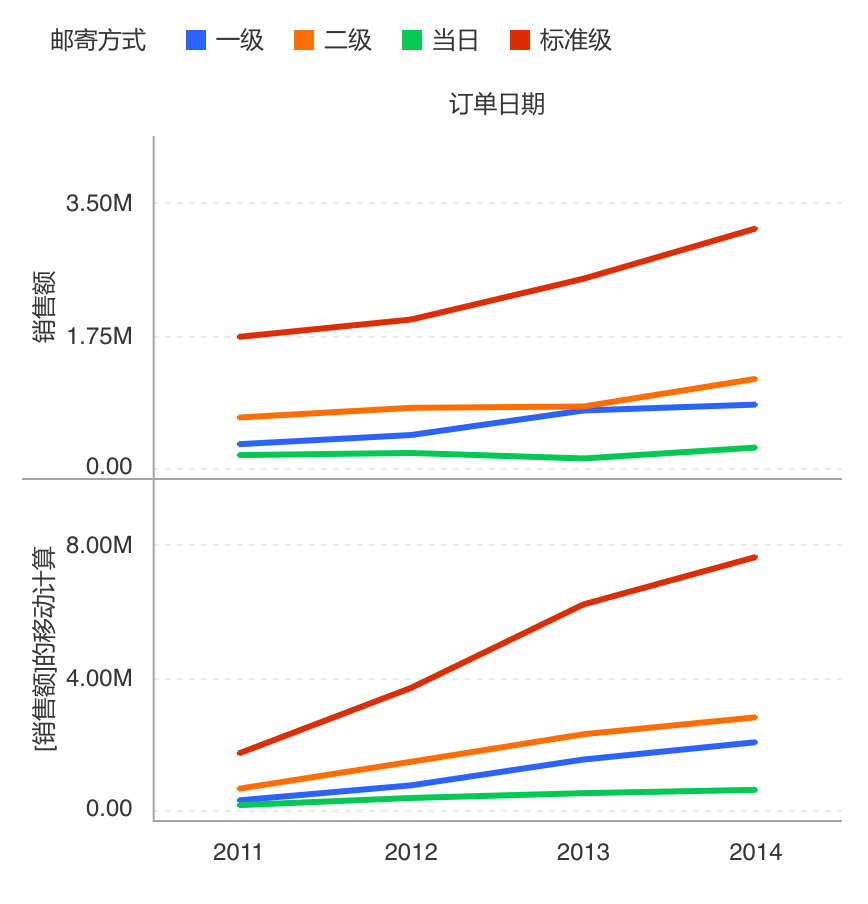## 排名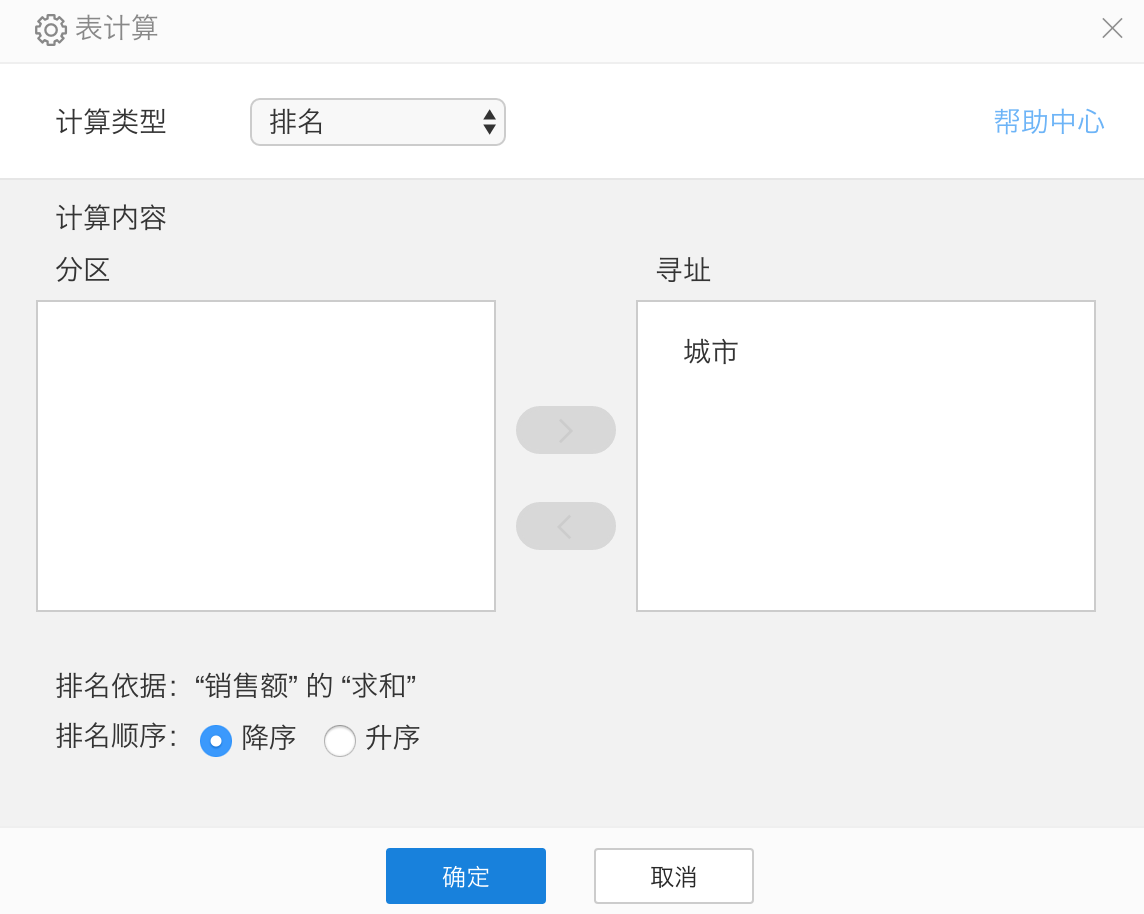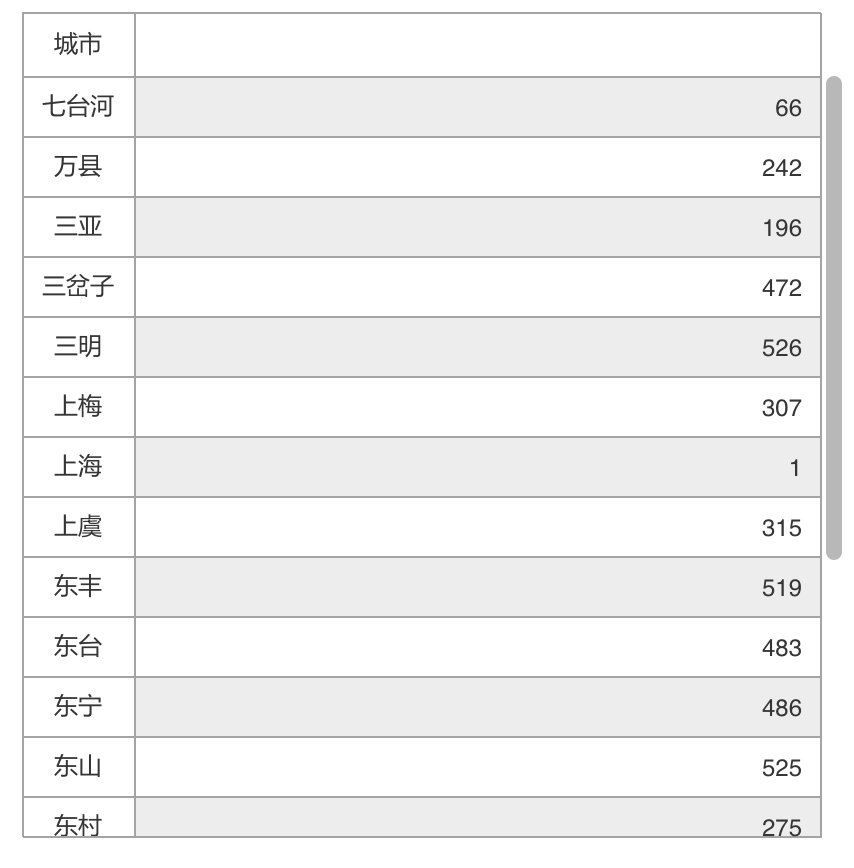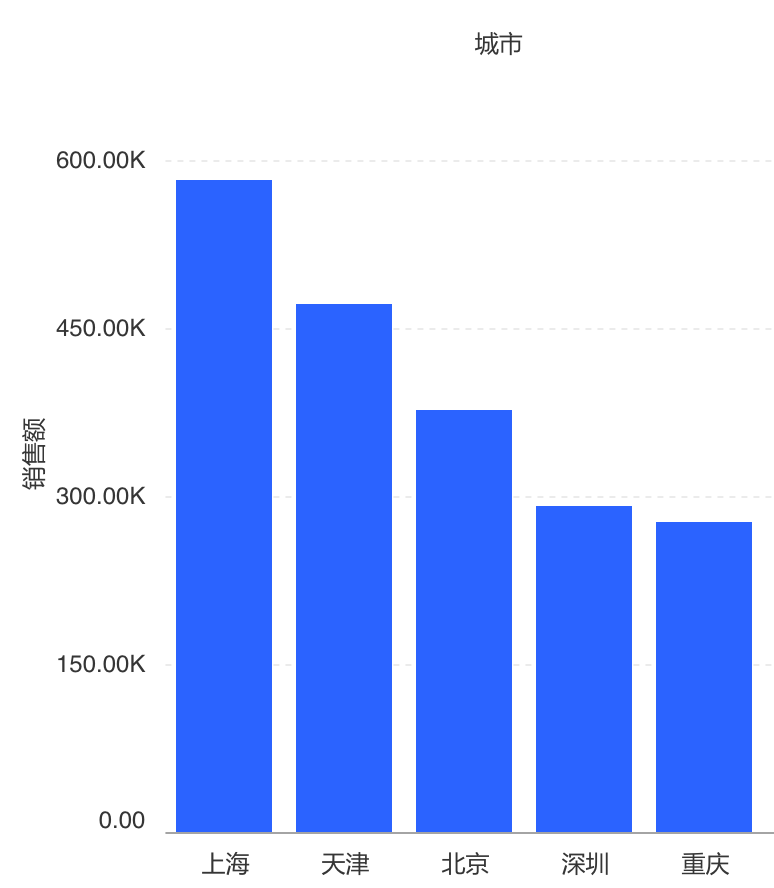## 表计算的动态计算方式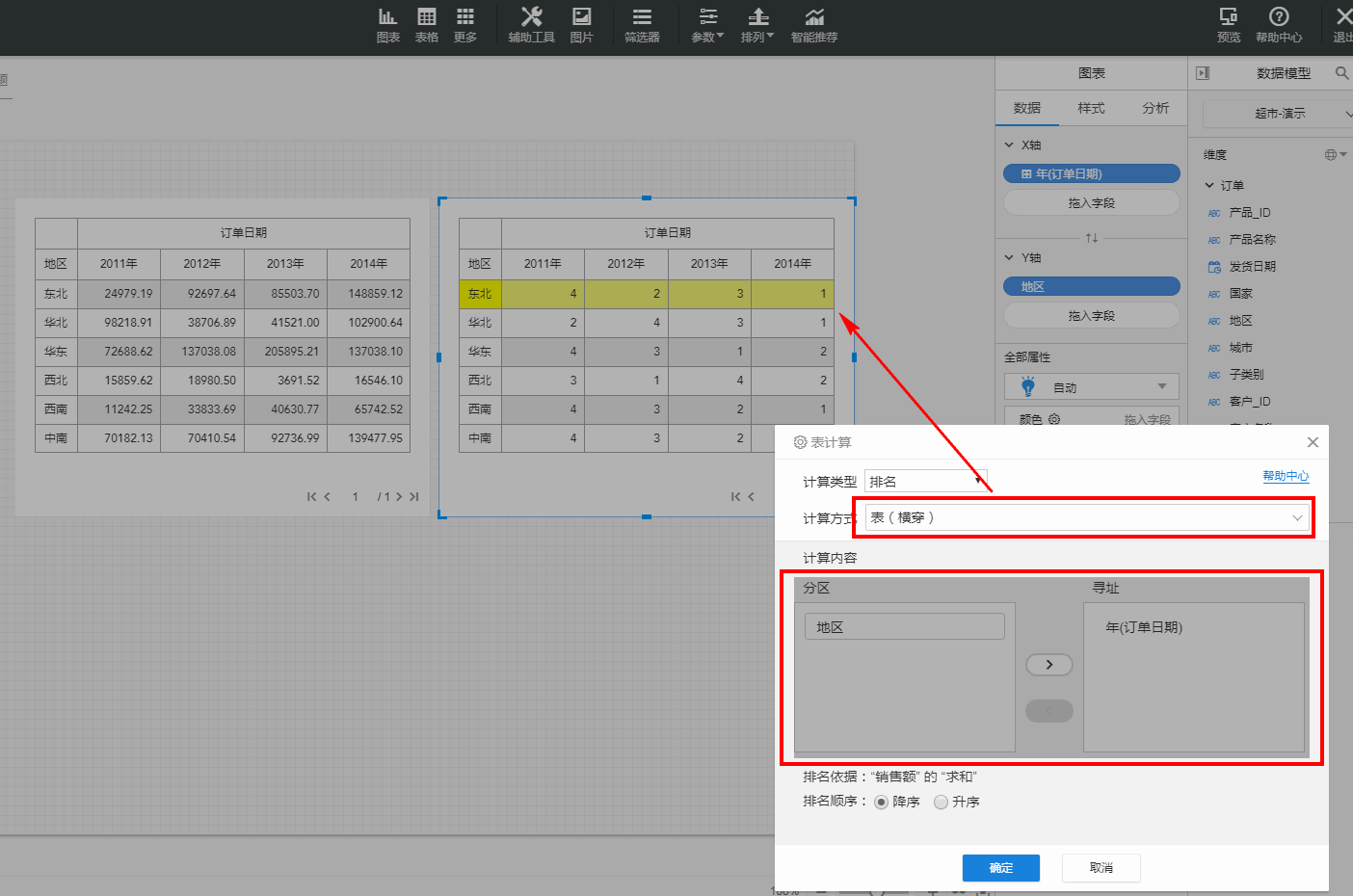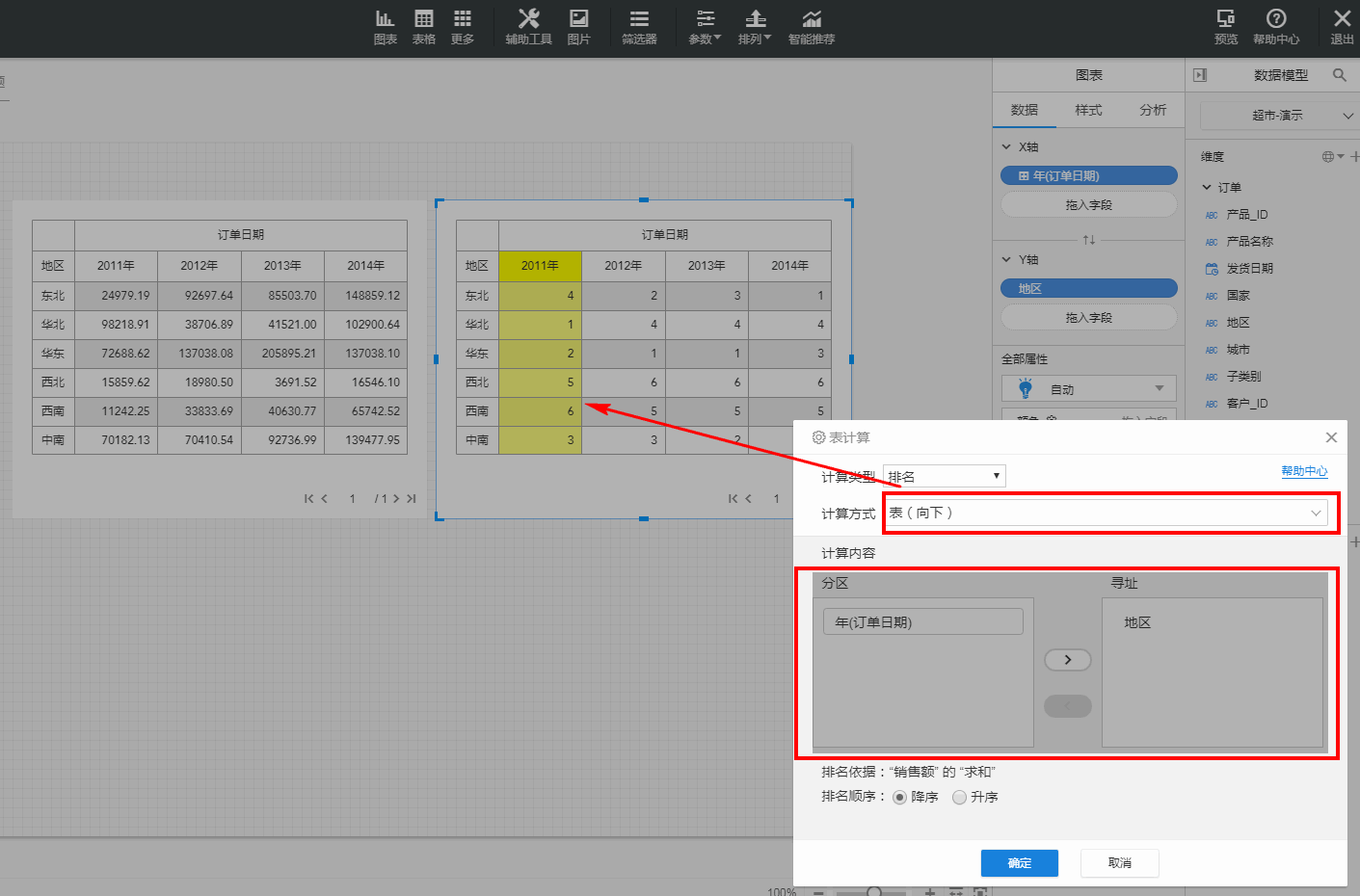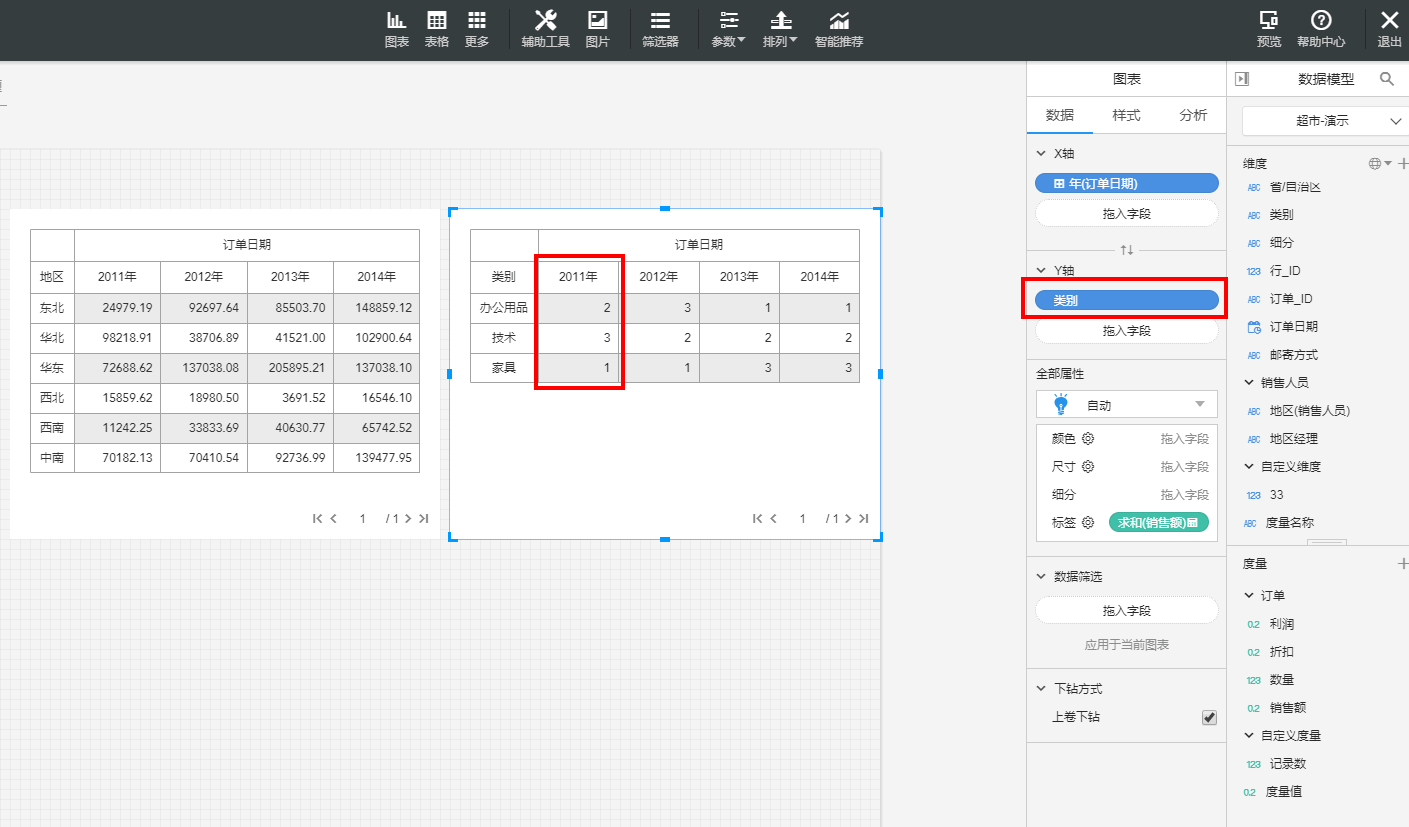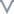根本没帮助文档较差文档一般文档不错文档很好

#### 更多建议

0/200